Courses

# White Noise Electronics and Communication Engineering (ECE) Notes | EduRev

## Communication Theory

Created by: Machine Experts

## Electronics and Communication Engineering (ECE) : White Noise Electronics and Communication Engineering (ECE) Notes | EduRev

The document White Noise Electronics and Communication Engineering (ECE) Notes | EduRev is a part of the Electronics and Communication Engineering (ECE) Course Communication Theory.
All you need of Electronics and Communication Engineering (ECE) at this link: Electronics and Communication Engineering (ECE)

WHITE NOISE:

White noise is a random signal (or process) with a flat power spectral density. In other words, the signal contains equal power within a fixed bandwidth at any center frequency. White noise draws its name from white light in which the power spectral density of the light is distributed over the visible band in such a way that the eye's three color receptors (cones) are approximately equally stimulated. In statistical sense, a time series rt is called a white noise if {rt} is a sequence of independent and identically distributed (iid) random variables with finite mean and variance. In particular, if rt is normally distributed with mean zero and variance σ , the series is called a Gaussian white noise.

An infinite-bandwidth white noise signal is a purely theoretical construction. The bandwidth of white noise is limited in practice by the mechanism of noise generation, by the transmission medium and by finite observation capabilities. A random signal is considered "white noise" if it is observed to have a flat spectrum over a medium's widest possible bandwidth.

WHITE NOISE IN A SPATIAL CONTEXT:

While it is usually applied in the context of frequency domain signals, the term white noise is also commonly applied to a noise signal in the spatial domain. In this case, it has an auto correlation which can be represented by a delta function over the relevant space dimensions. The signal is then "white" in the spatial frequency domain (this is equally true for signals in the angular frequency domain, e.g., the distribution of a signal across all angles in the night sky)'

STATISTICAL PROPERTIES:

The image to the right displays a finite length, discrete time realization of a white noise process generated from a computer.

Being uncorrelated in time does not restrict the values a signal can take. Any distribution of values is possible (although it must have zero DC components). Even a binary signal which can only take on the values 1 or -1 will be white if the sequence is statistically uncorrelated. Noise having a continuous distribution, such as a normal distribution, can of course be white.

It is often incorrectly assumed that Gaussian noise (i.e., noise with a Gaussian amplitude distribution — see normal distribution) is necessarily white noise, yet neither property implies the other. Gaussianity refers to the probability distribution with respect to the value i.e. the probability that the signal has a certain given value, while the term 'white' refers to the way the signal power is distributed over time or among frequencies.

We can therefore find Gaussian white noise, but also Poisson, Cauchy, etc. white noises. Thus, the two words "Gaussian" and "white" are often both specified in mathematical models of systems. Gaussian white noise is a good approximation of many real-world situations and generates mathematically tractable models. These models are used so frequently that the term additive white Gaussian noise has a standard abbreviation: AWGN. Gaussian white noise has the useful statistical property that its values are independent (see Statistical independence).

White noise is the generalized mean-square derivative of the Wiener process or Brownian motion.

APPLICATIONS:

It is used by some emergency vehicle sirens due to its ability to cut through background noise, which makes it easier to locate.

White noise is commonly used in the production of electronic music, usually either directly or as an input for a filter to create other types of noise signal. It is used extensively in audio synthesis, typically to recreate percussive instruments such as cymbals which have high noise content in their frequency domain.

It is also used to generate impulse responses. To set up the equalization (EQ) for a concert or other performance in a venue, a short burst of white or pink noise is sent through the PA system and monitored from various points in the venue so that the engineer can tell if the acoustics of the building naturally boost or cut any frequencies. The engineer can then adjust the overall equalization to ensure a balanced mix.

White noise can be used for frequency response testing of amplifiers and electronic filters. It is not used for testing loudspeakers as its spectrum contains too great an amount of high frequency content. Pink noise is used for testing transducers such as loudspeakers and microphones. White noise is a common synthetic noise source used for sound masking by a tinnitus masker.

White noise is a particularly good source signal for masking devices as it contains higher frequencies in equal volumes to lower ones, and so is capable of more effective masking for high pitched ringing tones most commonly perceived by tinnitus sufferers

White noise is used as the basis of some random number generators. For example, Random.org uses a system of atmospheric antennae to generate random digit patterns from white noise.

White noise machines and other white noise sources are sold as privacy enhancers and sleep aids and to mask tinnitus. Some people claim white noise, when used with headphones, can aid concentration by masking irritating or distracting noises in a person's environment.

MATHEMATICAL DEFINITION:

White random vector:

A random vector is a white random vector if and only if its mean vector and autocorrelation matrix are the following: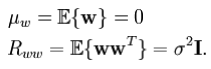That is, it is a zero mean random vector, and its autocorrelation matrix is a multiple of the identity matrix. When the autocorrelation matrix is a multiple of the identity, we say that it has spherical correlation.

White random process (white noise)

A continuous time random process w(t) where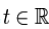is a white noise process if and only if its mean function and autocorrelation function satisfy the following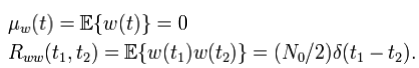i.e. it is a zero mean process for all time and has infinite power at zero time shift since its autocorrelation function is the Dirac delta function. The above autocorrelation function implies the following power spectral density.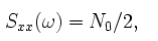since the Fourier transform of the delta function is equal to 1. Since this power spectral density is the same at all frequencies, we call it white as an analogy to the frequency spectrum of white light. A generalization to random elements on infinite dimensional spaces, such as random fields, is the white noise measure.

Random vector transformations

Two theoretical applications using a white random vector are the simulation and whitening of another arbitrary random vector. To simulate an arbitrary random vector, we transform a white random vector with a carefully chosen matrix. We choose the transformation matrix so that the mean and covariance matrix of thetransformed white random vector matches the mean and covariance matrix of the arbitrary random vector that we are simulating. To whiten an arbitrary random vector, we transform it by a different carefully chosen matrix so that the output random vector is a white random vector. These two ideas are crucial in applications such as channel estimation and channel equalization in communications and audio. These concepts are also used in data compression.

Simulating a random vector

Suppose that a random vector  X has covariance matrix Kxx. Since this matrix is Hermitian symmetric and positive semi definite, by the spectral theorem from linear algebra, we can diagonalize or factor the matrix in the following way.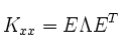where E is the orthogonal matrix of eigenvectors and Λ is the diagonal matrix of eigenvalues. We can simulate the 1st and 2nd moment properties of this random vector with mean and covariance matrix Kxx via the following transformation of a white vector  of unit variance: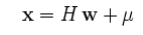where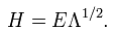Thus, the output of this transformation has expectation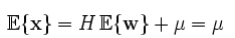and covariance matrix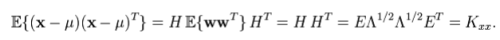Whitening a random vector

The method for whitening a vector X with mean μ and covariance matrix Kxx is to perform the following calculation: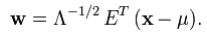Thus, the output of this transformation has expectation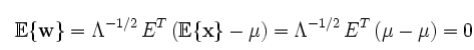and covariance matrix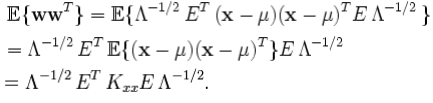By diagonalizing Kxx, we get the following: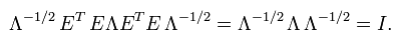Thus, with the above transformation, we can whiten the random vector to have zero mean and the identity covariance matrix.

Random signal transformations

We cannot extend the same two concepts of simulating and whitening to the case of continuous time random signals or processes. For simulating, we create a filter into which we feed a white noise signal. We choose the filter so that the output signal simulates the 1st and 2nd moments of any arbitrary random process. For whitening, we feed any arbitrary random signal into a specially chosen filter so that the output of the filter is a white noise signal.

Simulating a continuous-time random signal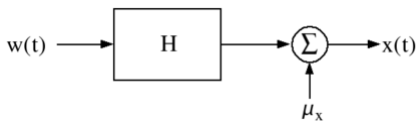White noise fed into a linear, time-invariant filter to simulate the 1st and 2nd moments of an arbitrary random process.

We can simulate any wide-sense stationary, continuous-time random process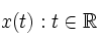with constant mean μ and covariance function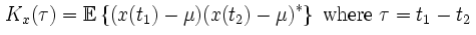and power spectral density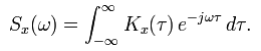We can simulate this signal using frequency domain techniques.

Because Kx(τ) is Hermitian symmetric and positive semi-definite, it follows that Sx(ω) is real and can be factored as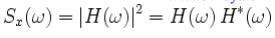if and only if Sx(ω) satisfies the Paley-Wiener criterion.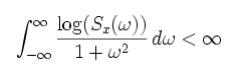If Sx(ω) is a rational function, we can then factor it into pole-zero form as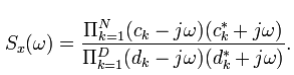Choosing a minimum phase H(ω) so that its poles and zeros lie inside the left half s-plane, we can then simulate x(t) with H(ω) as the transfer function of the filter.

We can simulate x(t) by constructing the following linear, time-invariant filter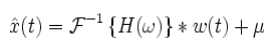where w(t) is a continuous-time, white-noise signal with the following 1st and 2nd moment properties: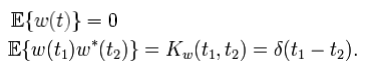Thus, the resultant signal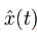has the same 2nd moment properties as the desired signal x(t)

Whitening a continuous-time random signal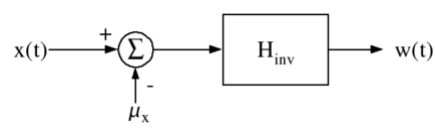An arbitrary random process x(t) fed into a linear, time-invariant filter that whitens x(t) to create white noise at the output.

Suppose we have a wide-sense stationary, continuous-time random process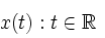defined with the same mean μ, covariance function Kx(τ), and power spectral density Sx(ω) as above.

We can whiten this signal using frequency domain techniques. We factor the power spectral density Sx(ω) as described above.

Choosing the minimum phase H(ω) so that its poles and zeros lie inside the left half s-plane, we can then whiten x(t) with the following inverse filter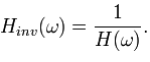We choose the minimum phase filter so that the resulting inverse filter is stable. Additionally, we must be sure that H(ω) is strictly positive for all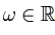so that Hinv(ω) does not have any singularities.

The final form of the whitening procedure is as follows: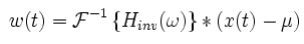so that w(t) is a white noise random process with zero mean and constant, unit power spectral density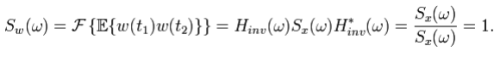Note that this power spectral density corresponds to a delta function for the covariance function of w(t)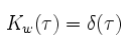Offer running on EduRev: Apply code STAYHOME200 to get INR 200 off on our premium plan EduRev Infinity!

,

,

,

,

,

,

,

,

,

,

,

,

,

,

,

,

,

,

,

,

,

;